# GSEB Solutions Class 11 Chemistry Chapter 3 Classification of Elements and Periodicity in Properties

Gujarat Board GSEB Textbook Solutions Class 11 Chemistry Chapter3 Classification of Elements and Periodicity in Properties Textbook Questions and Answers.

## Gujarat Board Textbook Solutions Class 11 Chemistry Chapter 3 Classification of Elements and Periodicity in Properties

### GSEB Class 11 Chemistry Classification of Elements and Periodicity in Properties Text Book Questions and Answers

Question 1.
What is the basic theme of organization in the periodic table?
It is to simplify and systematize the study of the properties of all elements. On the basis of similarities in chemical properties, the various elements have now been divided into different groups. Thus the basic theme of “organization in the periodic classification of elements is the electronic configuration of their atoms and their chemical properties based on the similarity in electronic configuration.Question 2.
Which important property did Mendeleev use to classify the elements in his periodic table and did he stick to that?
Mendeleev used the atomic weights of the elements as the main property to classify the elements. He arranged elements in horizontal rows and vertical columns of a table in order to their – increasing atomic weights in such a way that the elements with similar properties occupied the same vertical column or group.

He realized that some of the elements did not fit in with his scheme of classification of the order of atomic weight was strictly followed. In such cases, he ignored the order of atomic weights. For example iodine with lower atomic weight (= 126.9) than that of tellurium (= 127.6) (Group VI) was placed in Group VII along with other halogens because of similarities in properties.

Question 3.
What is the basic difference in approach between Mendeleev’s Periodic Law and the Modem Periodic Law?
Mendeleev’s Periodic Law was based upon atomic weights of elements, whereas Modem Periodic Law is based upon atomic numbers of elements.
Mendeleev stated: “The properties of the elements are a periodic function of their atomic weights”.
Modern Periodic Law states: “The physical and chemical properties of the elements are periodic functions of their atomic numbers”.

Question 4.
On the basis of quantum numbers justify that the sixth period of the periodic table should have 32 elements.
When n = 6, l = 0, 1, 2, 3, 4, 5. The order in which the energy of the orbitals increases is 6s < 4f< 5d < 6p. The total number of orbitals available are 16[s = 1, p = 3, d = 5, f= 7]. The maximum number of electrons which an orbital can contain is 2. Therefore the sixth period can accommodate 32 elements.Question 5.
In terms of period and group, where would you locate the element with Z = 114?
Since Z = 114; Its probable symbol is Uuq. It will lie in the 7th period and 14th group. Its structure will be 7s27p2.

Question 6.
Write the atomic number of the element present in the third period and a seventeenth group of the periodic table.
The atomic number of the element present in the third period and the seventeenth group is 17[2, 8, 7]. It is Chlorine.

Question 7.
Which element do you think would have been named by

1. Lawrence Berkeley Laboratory
2. Seaborg’s group?

1. Berkelium (Bk) with atomic number 97 and Lawrencium (Lr) with Z = 103
2. Seaborgium (Sg) with atomic number 106.

Question 8.
Why do elements in the same group have similar physical and chemical properties?
Elements in the same vertical column or group have similar. valence shell electronic configurations have the same number of electrons in the outer orbitals hence similar properties.
Thus the similarities in properties are due to similarities in the electronic configuration of the valence shells of atoms of the elements. For example, the Group I elements (alkali-metals) all have Is valence shell electronic configuration.

Question 9.
In, the case of non-metals like chlorine atomic radius is, actually covalent radius and it is half the bond distance between 2 atoms in chlorine molecule Cl2.
Bond distance in Cl2 molecule = 198 pm
Atomic or covalent radius =$$\frac { 198 }{ 2 }$$= 99 pm.

In the case of metals, atomic radius is actually metallic radius which is taken as half the internuclear distance separating the metal ions in the metallic crystal.

For example, the distance between two adjacent copper atoms in solid copper is 256 pm; hence the metallic radius (or atomic radius) of copper is 128 pm. On the other hand, the ionic radius is the radius of its ion (cation or anion). It is actually estimated by measuring the distances between cations and anions in ionic crystals.Question 10.
How does atomic radius vary in a period and in a group? How do you explain the variation?
Variation of Atomic radius across a period: Atomic radii of elements generally decrease in going from left to right in a period. It is because, within the same period, the outer electrons are in the same valence shell, and the effective nuclear charge increases as the atomic number increases resulting in the increased attraction of electrons to the nucleus.

Variation of Atomic radius within a group Atomic radii of elements within a group increase from top to bottom-In moving down a group, the nuclear charge increases with an increase in atomic number but at the same time there is a progressive increase in the principal quantum level, the number of electrons in the outermost shell, however, remains the same. Consequently, the distance of the outermost electrons from the nucleus gradually increases down the group because the effect of additional energy level is more pronounced than the effect of increased nuclear charge.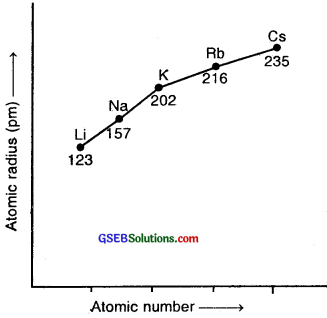Question 11.
What do you understand by isoelectronic species? Name a species that will be isoelectronic with each of the following atoms or ions.

1. F
2. Ar
3. Mg2+
4. Rb+

Isoelectronic species (atoms or ions) are those which contain the same number of electrons. For example, O2-, F, Na+, Mg2+ have 10 electrons each. They constitute isoelectronic series.

1. F is isoelectronic with Na+ (10e)
2. Ar is isoelectronic with K+ (18e)
3. Mg2+ is isoelectronic with O2- (10e)
4. Rb+ is isoelectronic with Br (36e)

Question 12.
Consider the following species :
N3-, O2-, F, Na+, Mg2+ and Al3+

1. What is common in them?
2. Arrange them in the order of increasing ionic radii.

1. The species N-3, O-2, F, Na+, Al3+ are all isoelectronic. containing 10 electrons each.
2. The species N-3, 0-2, F, Na+, Mg2+, Al3+ in the increasing order of ionic radii are Al3+ < Mg2+ <Na+ < F < O-2< N-3Question 13.
Explain why cations are smaller and anions larger in radii than their parent atoms?
Cations are always smaller than their parent atoms, because they have fewer electrons, while their nuclear charge remains the same. The remaining electrons are held more tightly by the protons in the nucleus and thus their radii are smaller than the parent atoms. Anions are always larger than their parent atoms because the addition of one or more electrons would result in increased repulsion among the electrons and a decrease in the effective nuclear charge.

Question 14.
What is the significance of the terms ‘isolated gaseous atom’ and ‘ground state’ while defining the ionization enthalpy and electron gain enthalpy?
While defining ionization enthalpy minimum energy is required to remove the most loosely bound electron from an isolated gaseous atom resulting in the formation of a positive ion
X (g) + energy → X+ (g) + e
while defining electron gain enthalpy, there is enthalpy change accompanying the process
X (g) + e → X (g) △H = △Heg
Again the energy is released when one electron is added to a neutral gaseous atom X to convert it into a negative ion. The atom must be present in the most stable state, i.e., the ground state. This is a requirement for comparison purposes.

Question 15.
The energy of an electron in the ground state of the hydrogen atom is -2.18 × 10-18 J. Calculate the ionization enthalpy of atomic hydrogen in terms of J mol-1
Solution:
A ground-state electron has E = – 2.18 × 10-18 J
The energy required for the removal of the electron is + 2.18 × 10-18 J
H (g) → H+ (g) + e △E = l.E. + 2.18 × 10-18 J/e
We have to apply the idea of mole concept:
In terms of per mole, it will be
2.18 × 10-18 J × 6.022 × 1023 = 13.12 × 105 J mol-1
[1 mole of electrons = 6.022 × 1023 electrons]

Question 16.
Among the second period elements the actual ionization enthalpies are in the order Li < B < Be.< C < O < N < F < Ne. Explain why

1. Be has higher △i H than B
2. Does O have lower △i H than N and F?

(1) △i H of Be is higher than that of B.
Electronic configuration of Be is 1s2, 2s2
whereas that of B is 1s2, 2s22px1
In the case of Be, the electron has to be removed from an s-orbital whereas, in the case of B, it has to be removed from the ap-orbital. It is difficult to remove an s-electron because it is closer to the nucleus than a p-electron hence more energy is required to remove an electron from 2s and Be than 2p-electron in the case of B. Hence IE of Be is higher than that of B

(2) Electronic configuration of O is.
1s2, 2s2, 2p2x 2p1y 2p1z. (neither exactly /half-filled nor completely filled)
whereas N is 1s2, 2s2, 2p2x 2p1y 2p1z(exactly half-filled)

It is difficult to remove an electron from the valence shell of N because its p-subshell is exactly half-filled and so has more stability whereas O has an electronic configuration that is neither completely filled nor exactly half-filled. Therefore, it is easier to remove one electron from an O atom. F, due to increased nuclear charge, has more IE than either O or N.Question 17.
How would you explain the fact that the first ionization enthalpy of sodium is lower than that of magnesium but its second ionization enthalpy is higher than that of magnesium?
The first electron in both cases has to be removed from 3s-orbital, but the nuclear charge of Na is less than that of Mg. Hence IE of Na is lower than that of Mg.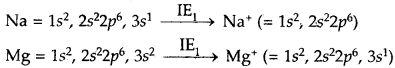After, the loss of the first electron the electronic configuration of Na+ is 1s2, 2s2,2p6 i.e., that of noble gas which is very stable, and hence the removal of the second electron from Na+ is very difficult. In the case of Mg+, after the loss of first electron, electronic configuration of Mg+ ion is + is 1s2, 2s2,2p6, 3s1. The second electron to be removed is from 3s orbital which is easier.
Hence IE2 of sodium is much larger than IE2 of Mg.

Question 18.
What are the various factors due to which the ionization enthalpy of the main group elements tends to decrease down a group?
(1) On moving down the group, the atomic size increases gradually due to the addition of one new principal energy shell at each succeeding element. As a result, the distance of the Valence electrons from the nucleus increases. Consequently, the force of attraction of. the nucleus for the valence electrons decreases and thus, IE should decrease.

(2) With the addition of new shells the number of inner shell electrons that shield the valence electrons from the nucleus increases. In other words, the shielding or screening effect increases. As a result, the force of attraction of the nucleus for the valence electrons further decreases, and hence the ionization enthalpy should decrease.

(3) Nuclear charge increases down the group with an increase in atomic number. As a result, the force of attraction of the nucleus for the valence electrons should increase thus, ionization enthalpy should increase.

The combined effect of the increase in the atomic size and the screening effect more than compensates, the effect of the increased nuclear charge. Consequently, the valence electrons become less and less firmly held by the nucleus hence the ionization enthalpies gradually decrease as we move down the group.Question 19.
The first ionization enthalpy values (in kJ mol-1) of group 13 elements are :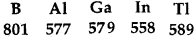How would you explain this deviation from the general trend?
On moving down the group 13 from B to Al, the first ionization enthalpies [IE1] decreases due to an increase in atomic size and screening effect which outweighs, the effect of increased nuclear charge. However, the IE1, of Ga is only slightly higher than that of Al [2 kJ mol-1] while that of TI is much higher than those of Al, Ga, and In.

This is due to the fact that Al follows immediately after s-block elements while Ga, and In follow after d-block elements and Tl after d- and f-block elements. These extra d and f electrons do not shield or screen the outer shell electrons from the nucleus effectively.

As a result, the valence shell electrons remain more tightly held by the nucleus, and hence larger amount of energy is required to remove them. This explains why Ga has higher IE1, than Al. In moving down from Ga to In, the increased shielding effect (due to the pressure of additional 4d-electrons) outweighs the effect of increased nuclear charge (49 – 31 = 18 units) and hence IE, of In is lower than that of Ga. Thereafter, the effect of increased nuclear charge (81 – 49 = 32 units) outweighs the shielding effect due to the presence of additional 4f and 5d electrons, and hence IE1, of Tl is higher than that of In.

Question 20.
Which of the following pairs of elements would have a more negative electron gain enthalpy?

1. O or F
2. F or Cl

1. F (- 328 kJ mol-1) has mere negative electron gain enthalpy than O (- 141 kJ mol-1).
2. Cl (- 349 KJ mol-1) has more negative electron gain enthalpy than F (- 328 kJ mol-1).

Question 21.
Would you expect the second electron gain enthalpy of O as positive, more negative, or Jess negative than the first? Justify your answer.
When an electron is added to an Oxygen atom, form O ion energy is released. But when another electron is added to O ion to form O2- ion energy is absorbed to overcome the strong electrostatic repulsion between the negatively charged O ion and the new electron being added. The second electron gain enthalpy of O is positive.
Thus, the first electron gain enthalpy :
O (g) + e (g) → O (g); △Heg = – 141 kJ mol-1 (energy is released)
Second electron gain enthalpy :
O (g) + e (g) → O2- (g) △Heg = + 780 kJ mol-1 (energy is absorbed)

Question 22.
What is the basic difference Ijetween the terms electron gain enthalpy and electronegativity?
Electronegativity differs from electrons, gain affinity in the sense that the latter is related to atoms in their isolated states, whereas the former is a property of an atom in a bonded state, i.e., in a molecule. Electronegativity is only qualitative and is not a measurable quantity.Question 23.
How would you react to the statement that the electronegativity of N on the Pauling scale is 3.0 in all the nitrogen compounds?
Though the value of electronegativity of N on the Pauling scale is 3.0, yet the electronegativity of any given element is not constant. It varies depending upon the element to which it is bound, its state of hybridization, and its oxidation state. The electronegativity increases as the %age of s-character of a hybrid orbital increase or the oxidation state of. the element increases.

The electronegativity of N in N02 where the oxidation state of N is + 4 is higher than in NO where the ox state of N is + 2. The value of electronegativity of 3.0 assigned to N is only arbitrary since it is not a measurable quantity.

Question 24.
Describe the theory associated with the radius of an atom as it

1. gains an electron
2. loses an electron

(1) When an atom gains one or more electrons, it becomes a negatively charged ion called an anion. The radius of an anion will be larger than that of its parent atom because the addition of one or more electrons would result in increased repulsion among the electrons and a decrease in effective nuclear charge. As a result electron cloud expands, i.e., ionic radius increases.

(2) When an atom loses one or more electrons it becomes a positively charged ion called cation The radius of a cation is always less than that of its parent atom. This may be due to the following reasons.

• The disappearance of the valence shell. In certain cases, the loss of electrons leads to a complete disappearance of the valence shell. Removal of the outermost shell decreases the size.
• Increase of effective nuclear charge. In a cation, the no of electrons is less than in the corresponding atom. The total nuclear charge remains the same. This increases the effective nuclear charge. As a result, the electrons are attracted towards the nucleus more tightly, thereby leading to a decrease in ionic radius.Question 25.
Would you expect the first ionization enthalpies of two isotopes of the same element to be the same or different? Justify your answer.
Isotopes of the same element differ only in the number of neutrons. Isotopes of the same element have the same no. of protons and electrons. IE values depend largely upon

1. nuclear charge
2. size of the atom IE does not depend upon the number of neutrons. Hence the value of IE remains the same for different isotopes of the same element.

Question 26.
What are the major differences between metals and non-metals?
Elements that have a strong tendency to lose electrons to form cations and have electropositive character are called metals while elements .which have a strong tendency to accept electrons and have electronegative character and form anions are called nonmetals. Therefore metals are strong reducing agents that have low ionization enthalpies, less negative electron gain enthalpies, low values of electronegativity. They form basic oxides and ionic compounds.

Non-Metals on the other hand, are strong oxidizing agents, have high ionization enthalpies, high electron gain enthalpies, the high value of electronegativity. They form acidic oxides and usually covalent compounds. Chemical differences between metals and non-metals are detailed below :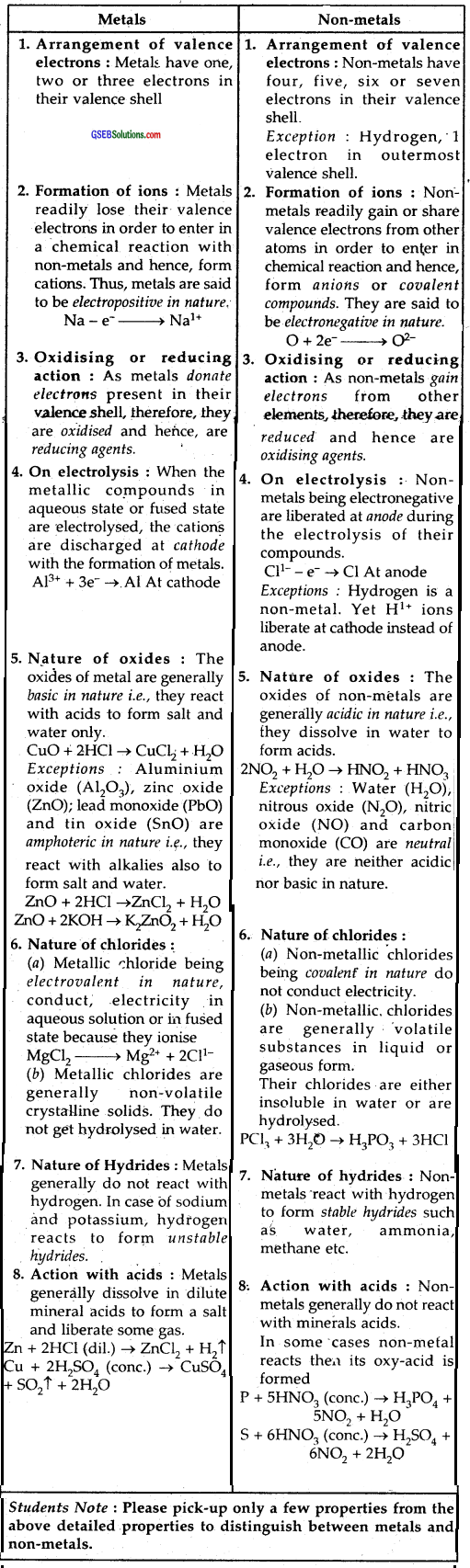Question 27.
Use the periodic table to answer the following questions.

1. Identify an element with five electrons in the outer subshell.
2. Identify an element that would tend to lose two electrons.
3. Identify an element that would tend to gain two electrons.
4. Identify the group having metal, non-metal, liquid as well as gas at room temperature.

1. Fluorine F = 1s2, 2s2, 2p5 [5e in 2p subshell]
2. Calcium Ca = 1s2, 2s2 2p6 3s2 3p6 4s2 [It can lose 2e]
3. Oxygen O = 1s2, 2s22p4 [It can gain 2e]
4. A metal which is a liquid at room temperature is mercury

(Hg). It is a transition metal and belongs to group 12. A non-metal which is a gas at room temperature is Nitrogen (N2) of group 15 or O2 of group 16 or F2/Cl2 of group 17 and inert gases (group 18). A non-metal that is liquid at room temperature is bromine (group 17).
The one group which has metal, non-metal, liquid as well as gas at room temperature is a group no. 1. It has H2 (gas at room temperature and a non-metal), Cs is a liquid – a metal.Question 28.
The increasing order of reactivity among group 1 elements is Li < Na < K < Rb <Cs whereas that among group 17 elements is F > Cl > Br > I. Explain.
The group I elements are alkali metals. They form unipositive ions M+ by losing one electron from their valence shells
M → M+ + e
As Ionisation enthalpy decrease’s from top to bottom in group I, the tendency to form unipositive ions increases from top to bottom.
Therefore the reactivity increases down the group from Li to Cs. In other words, the order of reactivity in group I elements is Li
< Na < K < Rb < Cs.
The group 17 elements aft halogens with an electronic configuration of ns2 np5 in their valence shells, try to become ns2np6 by acquiring one electron.
All the halogens are highly reactive elements due to the following two reasons.
(1) Low bond dissociation energy : (X2 → 2X) Halogens have low bond dissociation energy. F2 has the lowest bond dissociation energy which makes it the most reactive.

(2) High Electron Affinity: Due to high electron enthalpy, these elements have a strong tendency to gain an electron and thus are highly reactive.

(3) High reduction potentials :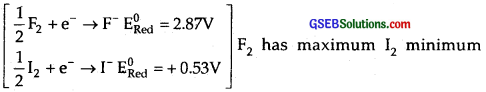reduction potentials. Hence order of reactivity is F > Cl > Br > I.

Question 29.
Write the general outer electronic configuration of s, p, d, and f-block elements.
The elements of s-block have outer electronic configuration of ns1 – 2. The elements of p-block have outer electronic configuration of
ns2np1 – 6 except He = 1s2
d-block elements have outer electronic configuration of (n – 1)d1 – 10 ns0 – 2
f-block elements have general outer electronic configuration of (n – 2) f0 – 14 (n – 1)d0 – 1 ns2

Question 30.
Assign the position of the element having outer electronic configuration

1. ns2np4 for n = 3
2. (n – 1)d2ns2 for n = 4, and
3. (n – 2)f7(n – 1)d1ns2 for n = 6, in the periodic table.

(1) ns2np4 is 3s23p4
The complete electronic configuration is
1s2, 2s22p6, 3s23p4
Atomic Number = 2+ 2 + 6 + 2 + 4 = 16
The element is sulphur in the 3rd period and in Group 16 (p- block)

(2) (n – 1) d2ns2 for n = 4;4t is 3d24s2
The complete electronic configuration is
1s2, 2s22p6, 3s23p63d24s2
Atomic number is 22, the element is Titanium.
It is a transition element present in the 4th period and Group 4.

(3) (n – 2)f7 (n – 1)d1 ns2 for n = 6
4f7 5d16s2
Its complete electronic configuration is 1s2, 2s2, 2p6, 3s2 3p6 3d10 4s2 4p6 4d10 4f7 5s25p65d16s2
It is an inner transition element, belongs to Lanthanoid series or 4fseries. It is an f-block element.

Question 31.
The first (△iH1) and the second (△1H2) ionization enthalpies (in kJ mol-1) and the (△eg H) electron gain enthalpy (in kJ mol-1)of a few elements are given below :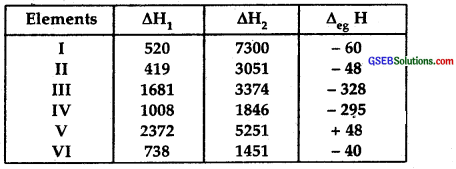Which of the above elements is likely to be :

1. the least reactive element,
2. the most reactive metal.
3. the most reactive non-metal
4. the least reactive non-metal.
5. the metal which can form a stable binary halide of the formula MX2 (X = hydrogen).
6. the metal which can form a predominantly stable covalent halide of the formula MX (X = halogen)?

(1) The least reactive element is V.
It is Helium. It has no tendency to lose electrons (as is clear from its high △H1 and its higher △H2), nor can it gain electrons (positive value of electron gain enthalpy – energy has to be absorbed to gain or add an electron)

(2) The most reactive metal is II, it is K.
Its △H1 is the lowest and △H2 is very high i:e., it can lose one electron readily from its valance shell (4th) to become K+. It cannot lose the 2nd electron readily because of the stable Ar core.

(3) The most reactive non-metal is III, it is Fluorine. Its △H1 and △H2 are very high, meaning it cannot lose electrons readily. On the other hand, its electron gain enthalpy has a large negative value indicating it can accept an electron readily.

(4) The least reactive non-metal is IV. It is iodine. Its △H1 and △H2 are very high, meaning it cannot lose electrons readily: On the other hand, its electron gain enthalpy has a large negative value (but not as large a negative value as F above) indicating that it can accept art electron readily.

(5) The metal which can form a stable binary halide of the formula MX2 (X = halogen) is VI. It is Magnesium
Mg + X2 → MgX2
Its △H1 and △H2 are not very high, but its electron gain enthalpy is very small △H2 is not very large as a compound to △H1
Because of its electropositive nature and tendency to lose two electrons, it can form a stable binary halide MX2.

(6) The metal which can form a stable covalent halide is I. It is Lithium its △H2> > △H1, meaning it can lose one electron readily but not the second. Due to its small size, it forms a stable covalent halide of the formula MX.Question 32.
Predict the formulas of the stable binary compounds that would be formed by the combination of the following pairs of elements.

1. Lithium and oxygen
2. Magnesium and nitrogen
3. Aluminum and iodine
4. Silicon and oxygen
5. Phosphorus and fluorine
6. Element Lu and fluorine

1. Lithium oxide — Li2O
2. Magnesium nitride — Mg3N2
3. Aluminum iodide — All3
4. Silicon oxide or silica — SiO2
5. Phosphorus fluoride — PF5
6. Element (Lu) fluoride — EF3 [LuF3]

Question 33.
In the modem periodic table, the period indicates the value of:

1. atomic number
2. atomic mass
3. principal quantum number
4. azimuthal quantum number

3. Principal quantum number.

Question 34.
Which of the following statements related to the modem periodic table is incorrect?

1. The p-block has 6 columns because a maximum of 6 electrons can occupy all the orbitals in a p-shell.
2. The d-block has 8 columns because a maximum of 8 electrons can occupy all the orbitals in a d-subshell.
3. Each block contains a number of columns equal to the number of electrons that can occupy that subshell.
4. The block indicates the value of an azimuthal quantum number (0 for the last subshell that received electrons in building up the electronic configuration.

2. the d-block has 10 columns because a maximum of 10 electrons can occupy all the five d-orbitals of the d-subshell.

Question 35.
Anything that influences the valence electrons will affect the chemistry of the element. Which one of the following factors does not affect the valence shell?

1. Valence principal quantum number (M)
2. Nuclear charge (Z) (c) Nuclear mass
3. A number of core electrons.

3. Nuclear mass does not affect the valence shell because the nucleus consists of protons and neutrons whereas protons, i.e., nuclear charge affects the valence shell, but neutrons do not. Hence option (c) is wrong.Question 36.
The size of isoelectronic species — F, Ne and Na+ is affected by

1. nuclear charge’ (Z)
2. valence principal quantum number (n)
3. electron-electron interaction in the outer orbitals
4. none of the factors because their size is the same.

1. Nuclear charge (Z).

Question 37.
Which one of the following statements is incorrect in relation to ionization enthalpy?

1. Ionization enthalpy increases for each successive electron.
2. The greatest increase in ionization enthalpy is experienced in the removal of electrons from the core noble gas configuration.
3. The end of valence electrons is marked by a big jump in ionization enthalpy.
4. Removal of electrons from orbitals bearing a lower n value is easier than from orbital having a higher n value.

4. the statement is incorrect. It is easier to remove an electron from orbitals bearing a higher n value than from orbital having a lower n value.

Question 38.
Considering the elements B, Al, Mg and K, the correct order of their metallic character is :

1. B > Al > Mg > K
2. Al > Mg > B > K
3. Mg > Al > K > B
4. K > Mg > Al > B

4. K > Mg > Al > B

Question 39.
Considering the elements B, C, N F and So, the correct order of their non-metallic character is:

1. B > C >Si > N > F
2. Si > C > B N > F
3. F > N > C > B > Si
4. F > N > C > Si > B

3. is correct.
In a period the non-metallic character increases from left to right. Thus among B, C, N, F the non-metallic character decreases in order.
F > N > C. > B. However within a group non-metallic character decreases from top to bottom. Thus C is more non-metallic than Si. Therefore the correct sequence of decreasing non-metallic character is F > N > C > B > Si.

Question 40
Considering the elements F, Cl, O and N, the correct order of their chemical reactivity in terms of oxidizing property is :

1. F > Cl > O > N
2. F > O > Cl > N
3. Cl > F > O > N
4. O > F > N > Cl